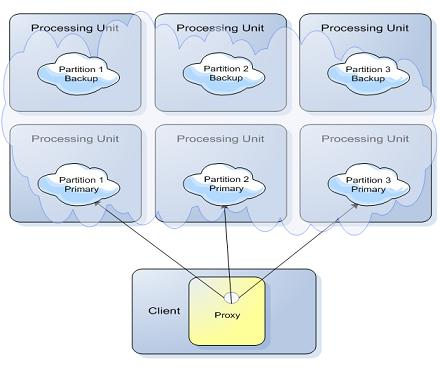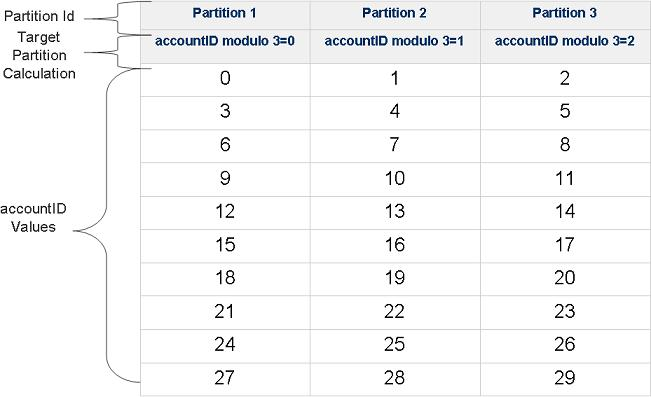This page describes an older version of the product. The latest stable version is 16.1.

# Data Partitioning

Load balancing (Data Partitioning) is essential in any truly scalable architecture, as it enables scaling beyond the physical resources of a single-server machine. In XAP, load balancing is the mechanism used by the clustered proxy to distribute space operations among the different cluster members. Each cluster member can run on a different physical or virtual machine.

A clustered proxy for a partitioned data grid holds logical references to all space members in the cluster. The references are “logical”, in the sense that no active connection to a space member is opened until it is needed. This is illustrated in the following diagram:This is the default mode and applicable for most of the application. When using hash-based load-balancing policy the client proxy spread new written space objects into relevant cluster space nodes. The relevant space to rout the operation is determined using space object routing field (aka also as routing index) value hash code. This value together with the number of the cluster partitions used to calculate the target space for the operation.

``````Target partition space ID = safeABS(routing field hashcode) % (# of partitions)

int safeABS( int value)
{
return value == Integer.MIN_VALUE ? Integer.MAX_VALUE : Math.abs(value);
}
``````

The routing field must implement the `hashCode` method and will be used both when performing write and read operations. When using this approach the assumption is there is normal distribution of routing field values to have even distribution of the data across all the cluster partitions.

Getting the number of partitions can be done via:

``````Admin admin = new AdminFactory().discoverUnmanagedSpaces().addLocator(locators).createAdmin();
int partitionCount = admin.getSpaces().waitFor("MyDataGridName", 5, TimeUnit.SECONDS).getPartitions().length;
``````
Tip
• It is recommended to use an Integer or a Long field type to be used as the routing field.
• Default routing field is the space id field. In such a case make sure you have the @SpaceId using the `autoGenarate=false` mode.
Important

Converting a numeric value to a String and using the string representation as the routing key will give a different load balancing in comparison to a numeric value.

# Example

A cluster is defined with 3 partitions where each partition has one primary and one backup space.The cluster configured to use the hash-based load-balancing policy. The application writes the `Account` space object into the clustered space. The `accountID` field as part of the `Account` class is defined as the routing field using the `@SpaceRouting` decoration.

The Account class implementation:

``````@SpaceClass
public class Account
{
Integer accountID;
String accountName;

@SpaceRouting
public Integer getAccountID()
{
return accountID;
}

public String getAccountName()
{
return accountName;
}
// setter methods
...
}
``````

The `accountID` field value is used by the space client proxy together with the number partitions in the following manner to determine the target space for the write, read, or take operations:

``````Target Space number = (accountID hashCode value) modulo (Partitions Amount)
``````

If we will write 30 Account space objects with different `accountID` values into the cluster, the space objects will be routed into the 3 partitions in the following manner:Tip

If the relevant target space or its backup space is not active an error message will be thrown.

See below Hash based Load-Balancing Calculator that calculates the target space of a given routing value. You may change this to use String based routing values or numerical values.

``````import java.util.Hashtable;
import java.util.Random;

int partitions = 10;

Random rand = new Random(1000);
int maxObject = 1000;
String values[] = new String[maxObject];
for (int i = 0; i < maxObject; i++) {
values[i] = String.valueOf(rand.nextInt(maxObject));
}

Hashtable<Integer, Integer> dist = new Hashtable<Integer, Integer>();
for (int i = 0; i < values.length; i++) {
int hc = values[i].hashCode();
int targetPartitionID = safeABS(hc) % partitions;
Integer dist_value = (Integer) dist.get(targetPartitionID);

if (dist_value == null)
dist_value = new Integer(0);

dist.put(targetPartitionID, dist_value.intValue() + 1);
}
System.out.println("Total amount of objects:" + maxObject);
System.out.println("Total amount of partitions:" + partitions);

for (int i = 0; i < dist.size(); i++) {
System.out.println("Partition " + i + " has " + dist.get(i)	+ " objects");
}
System.out.println();
System.out.println("Routing field values:");
for (int i = 0; i < maxObject; i++) {
System.out.print(values[i] + ",");
if ((i % 80 == 0) && (i > 80))
System.out.println();
}
}

static int safeABS(int value) {
return value == Integer.MIN_VALUE ? Integer.MAX_VALUE : Math.abs(value);
}
``````

Here is an example output:

``````Total amount of objects:1000
Total amount of partitions:10
Partition 0 has 107 objects
Partition 1 has 104 objects
Partition 2 has 104 objects
Partition 3 has 99 objects
Partition 4 has 104 objects
Partition 5 has 92 objects
Partition 6 has 103 objects
Partition 7 has 103 objects
Partition 8 has 90 objects
Partition 9 has 94 objects

Routing field values:
487,935,676,124,....
``````

# Batch Operation Execution Mode

The following table specifies when the different batch operations executed in parallel manner and when in serial manner when the space running in partitioned mode:

Operation Transactional Max values Execution Mode Returns when..
readMultiple NO n/a Parallel Returns when all spaces completed their operation
readMultiple YES Integer.MAX_VALUE Serial Returns when found enough matching space objects
readMultiple n/a Integer. MAX_VALUE Parallel Returns when all spaces completed their operation
takeMultiple n/a Integer.MAX_VALUE Serial Returns when all spaces completed their operation
takeMultiple n/a Integer.MAX_VALUE Parallel Returns when all spaces completed their operation
writeMultiple n/a n/a Parallel Returns when all spaces completed their operation
updateMultiple n/a n/a Parallel Returns when all spaces completed their operation
• A Parallel operation on the client means that the partition proxy will run a thread against each of its constituent partition proxies, each thread executing the operation (take , read). Corollary: every partition will experience a read/take multiple. No partition can guarantee that it will supply objects that the application client obtains in its result.
• A Serial operation on the client means that the partition proxy will execute one read/take multiple on each partition, the partitions being accessed in partition number order, 0 through max partition number. The readMultiple/takeMultiple will complete as soon as the requisite number of objects has been read/taken, or all partitions accessed, whichever comes soonest. Corollary: only partition 0 can guarantee that it will experience a readMultiple/takeMultiple - i.e. according to the member ID - Each partition has a numeric running ID associated with it and the processing done according their order(0 to N).
• When executing custom queries against partitioned space (triggers the space filter) you should use the takeMultiple or readMultiple with Integer.MAX_VALUE as the max values to execute the filter operation in parallel across the partitions.
• WriteMultipe/UpdateMultiple with a transaction executed in parallel manner.
• When calling any of the batch operations with a template and set the routing id in the template (making sure all the space objects go to the same physical space in a cluster) you do not need to use jini transaction. Local transaction will suffice.
• readMultiple/takeMultiple against any single partition is a synchronous call in the associated partition specific client proxy. The space operation is a synchronous operation. It will return as many requested objects as are currently present in the space, up to the number requested. If no objects are matched an empty array is returned.

# Considerations

• The replication scheme does not take into account `IReplicatable` (partial replication) and replication matrix.
• All objects with a given routing value will be stored in the same partition. This means that a given partition must be able to hold all similarly-routed objects. If the routing value does not have uniform distribution the partitioning will be uneven. Use a derived routing field (such as ID field) as the routing field value that gives a flat distribution across all nodes, if possible.